# How to Calculate and Solve for Resiliency | Fracture Mechanics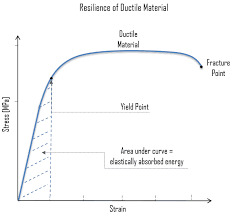The image above represents resiliency.

To compute for resiliency, two essential parameters are needed and these parameters are Maximum Stress (σmax) and Young’s Modulus of Elasticity (E).

The formula for calculating resiliency:

R = σmax² / 2E

Where:

R = Resiliency
σmax = Maximum Stress
E = Young’s Modulus of Elasticity

Let’s solve an example;
Find the resiliency when the maximum stress is 32 and the young’s modulud of elasticity is 12.

This implies that;

σmax = Maximum Stress = 32
E = Young’s Modulus of Elasticity = 12

R = σmax² / 2E
R = 32² / 2(12)
R = 1024 / 24
R = 42.67

Therefore, the resiliency is 42.67 Pa.

Calculating the Maximum Stress when the Resiliency and the Young’s Modulus of Elasticity is Given.

σmax = R x 2E

Where;

σmax = Maximum Stress
R = Resiliency
E = Young’s Modulus of Elasticity

Let’s solve an example;
Find the maximum stress when the resiliency is 15 and the young’s modulus of elasticity is 3.

This implies that;

R = Resiliency = 15
E = Young’s Modulus of Elasticity = 3

σmax = R x 2E
σmax = 15 x 2 x 3
σmax = 90
σmax = 9.48

Therefore, the maximum stress is 9.48.

Calculating the Young’s Modulus of Elasticity when the Resiliency and the Maximum Stress is Given.

E = σmax² / R X 2

Where;

E = Young’s Modulus of Elasticity
R = Resiliency
σmax = Maximum Stress

Let’s solve an example;
Find the young’s modulus of elasticity when the resiliency is 18 and the maximum stress is 48.

This implies that;

R = Resiliency = 18
σmax = Maximum Stress = 48

E = σmax² / R X 2
E = 48² / 18 X 2
E = 2304 / 36
E = 64

Therefore, the young’s modulus of elasticity is 64.

Nickzom Calculator – The Calculator Encyclopedia is capable of calculating the resiliency.

To get the answer and workings of the resiliency using the Nickzom Calculator – The Calculator Encyclopedia. First, you need to obtain the app.

You can get this app via any of these means:

To get access to the professional version via web, you need to register and subscribe for NGN 2,000 per annum to have utter access to all functionalities.
You can also try the demo version via https://www.nickzom.org/calculator

Android (Paid) – https://play.google.com/store/apps/details?id=org.nickzom.nickzomcalculator
Android (Free) – https://play.google.com/store/apps/details?id=com.nickzom.nickzomcalculator
Apple (Paid) – https://itunes.apple.com/us/app/nickzom-calculator/id1331162702?mt=8
Once, you have obtained the calculator encyclopedia app, proceed to the Calculator Map, then click on Materials and Metallurgical under Engineering.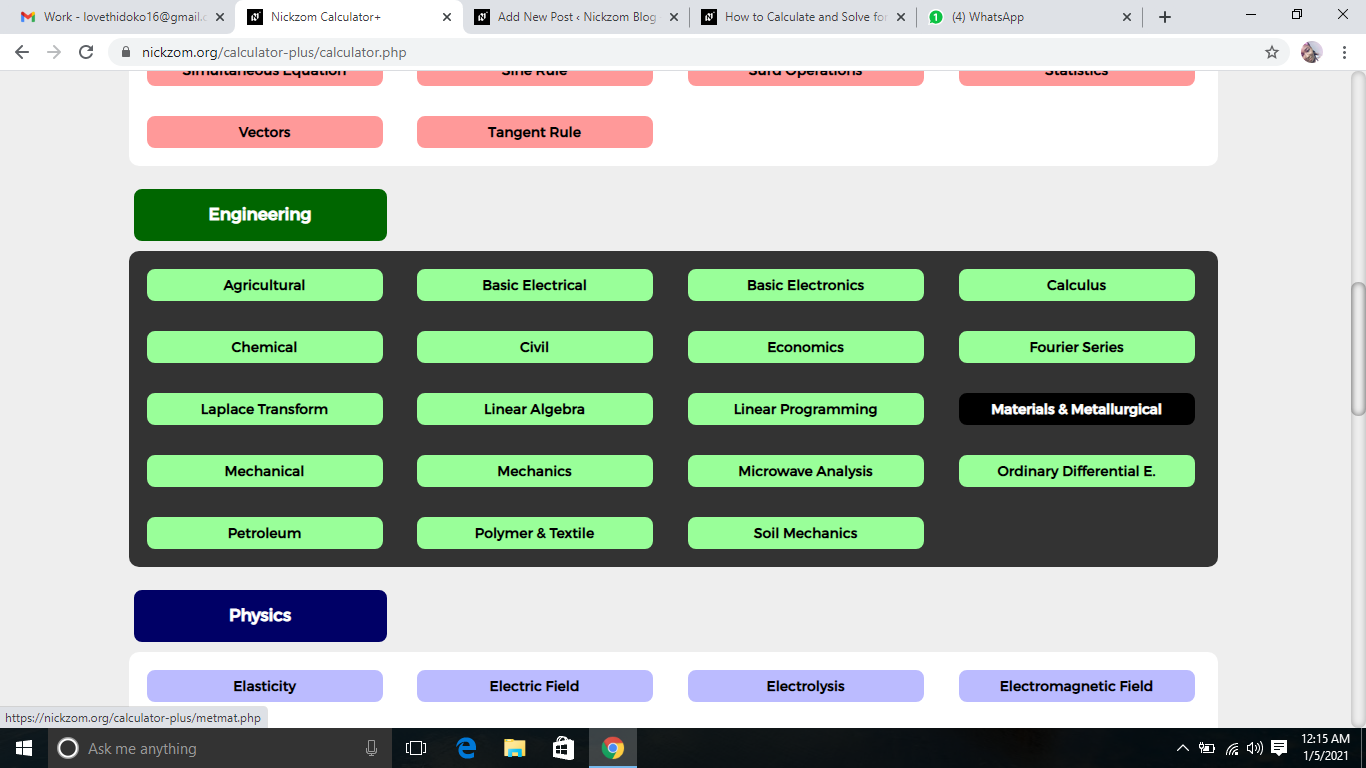Now, Click on Fracture Mechanics under Materials and MetallurgicalNow, Click on Resiliency under Fracture Mechanics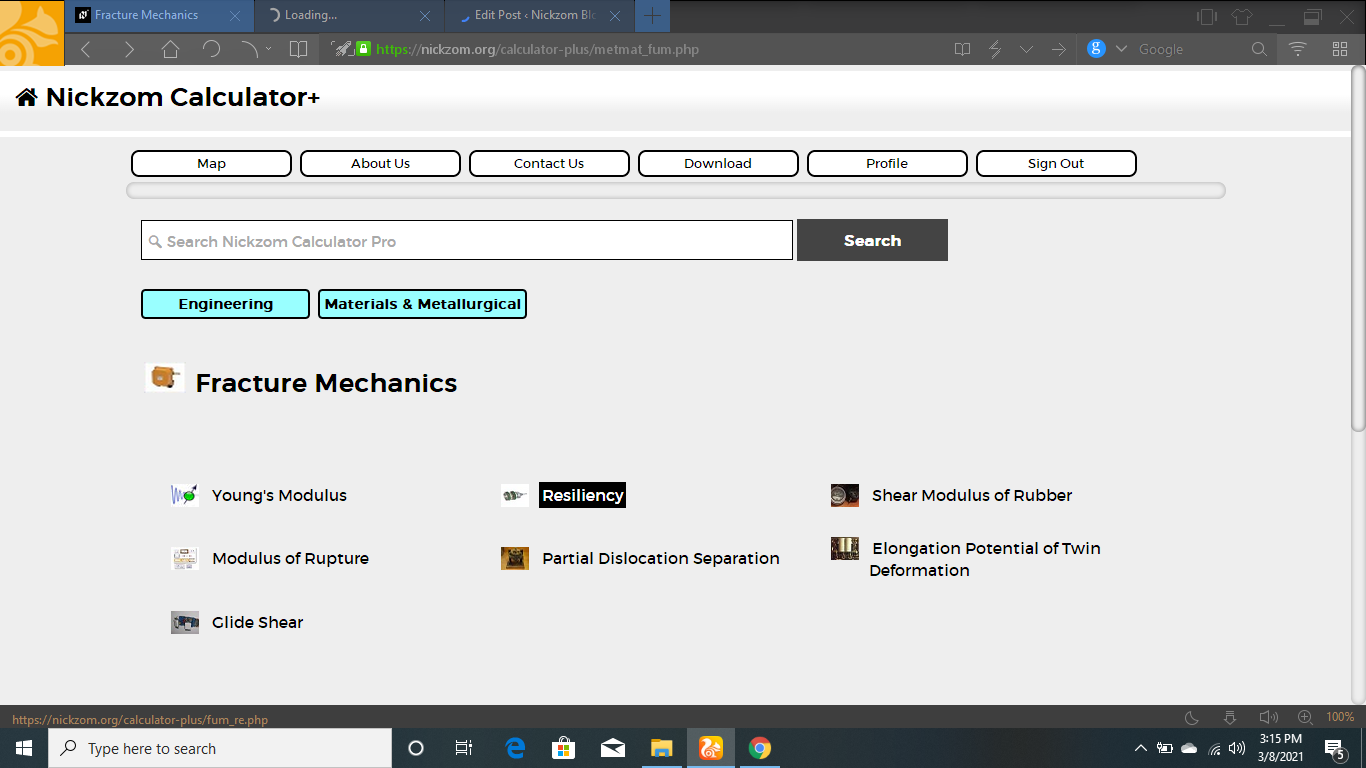The screenshot below displays the page or activity to enter your values, to get the answer for the resiliency according to the respective parameters which is the Maximum Stress (σmax) and Young’s Modulus of Elasticity (E).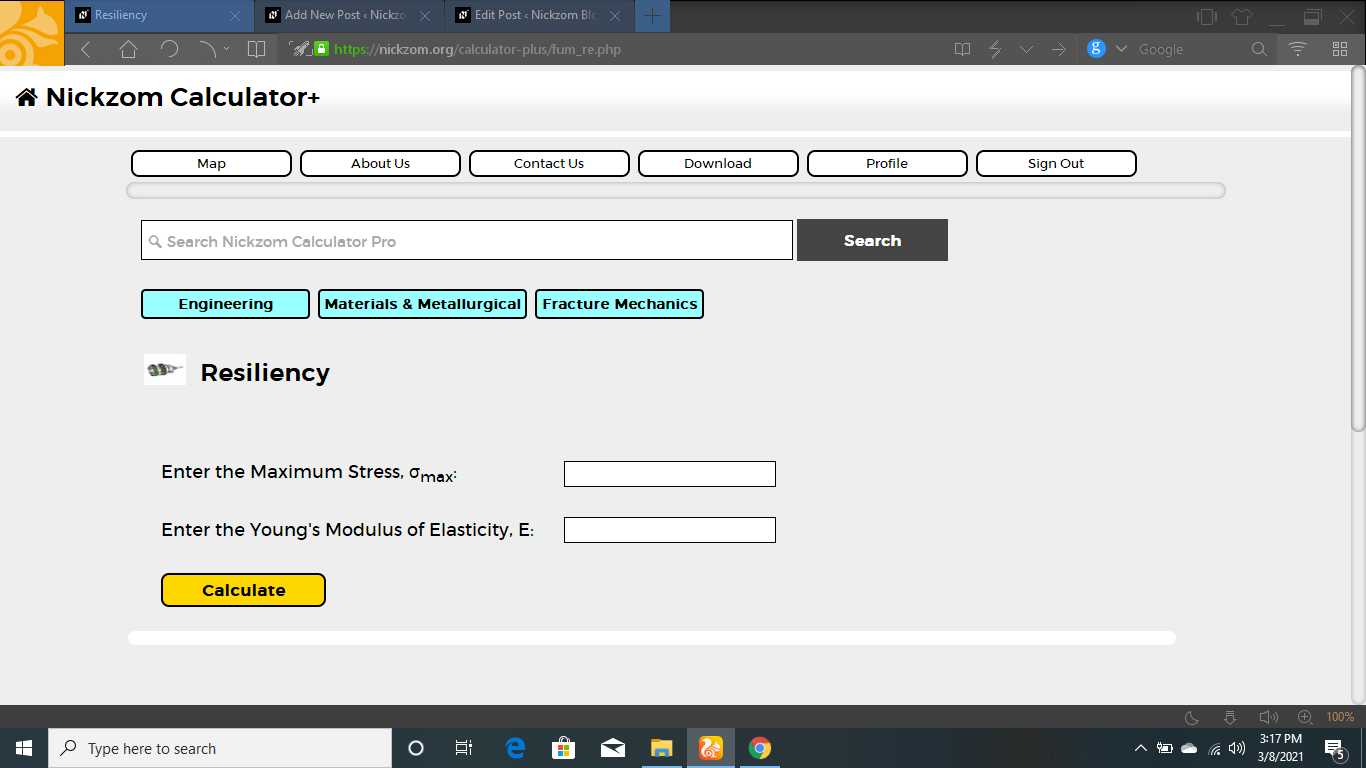Now, enter the value appropriately and accordingly for the parameter as required by the Maximum Stress (σmaxis 32 and Young’s Modulus of Elasticity (E) is 12.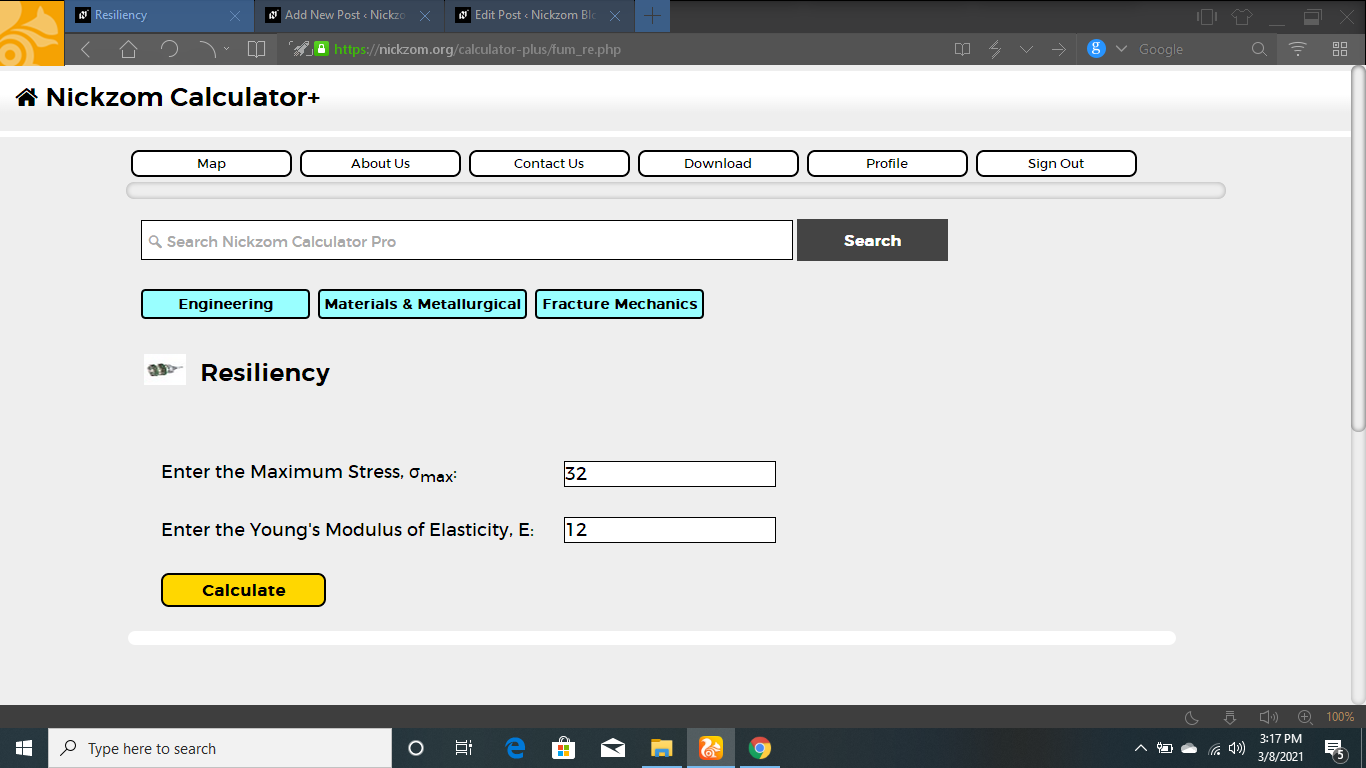Finally, Click on Calculate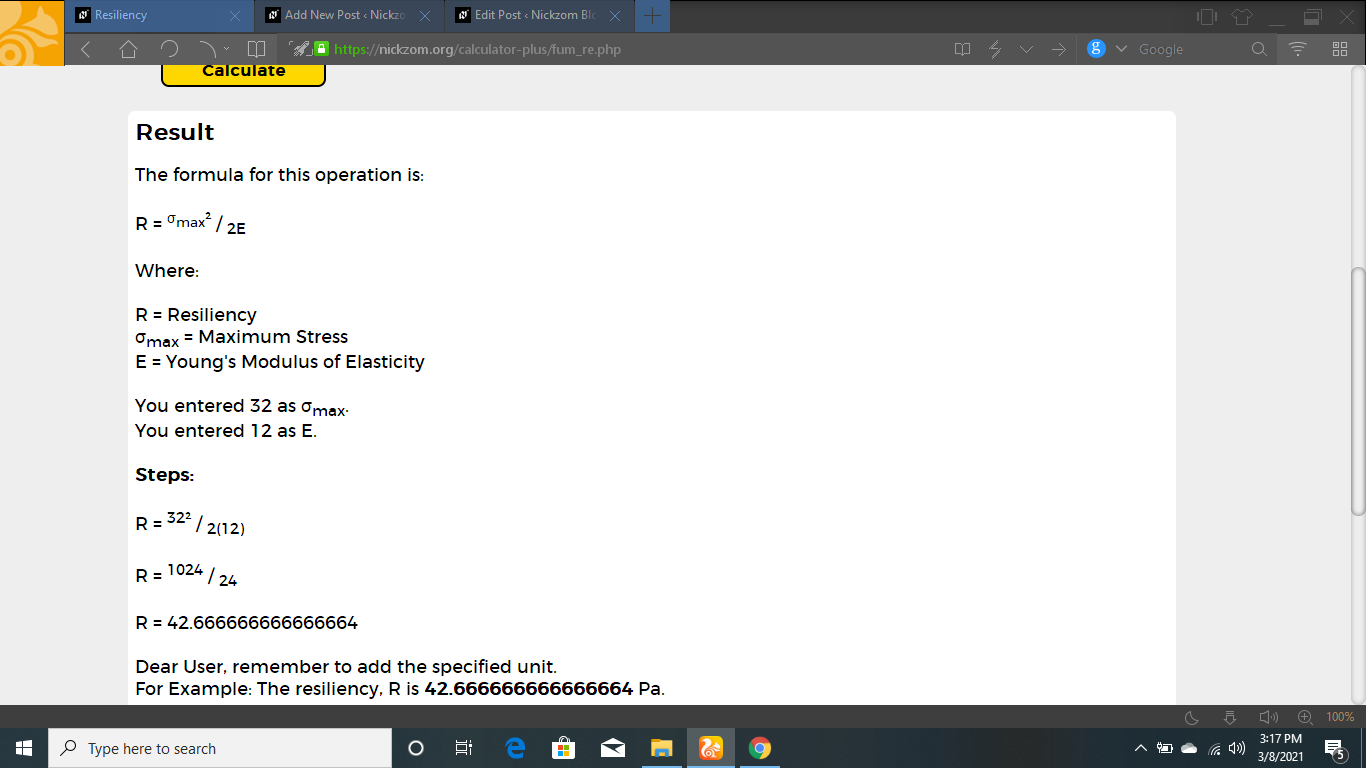As you can see from the screenshot above, Nickzom Calculator– The Calculator Encyclopedia solves for the resiliency and presents the formula, workings and steps too.